# Wooden container

The locksmith should cover the cube-shaped wooden container with a metal sheet inside. The outer edge of the container is 54cm. The wall thickness is 25 mm. The container has no lid. Calculate. How many sheets will be needed to cover it?

S =  12495 cm2

### Step-by-step explanation: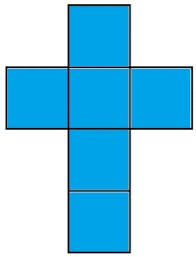Did you find an error or inaccuracy? Feel free to write us. Thank you!Tips to related online calculators
Do you want to convert area units?
Do you want to convert length units?

#### You need to know the following knowledge to solve this word math problem:

We encourage you to watch this tutorial video on this math problem:

## Related math problems and questions:

• Metal sheet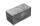How much m ^ 2 of sheet metal is needed to beat a box in the shape of a cube with an edge length of 50 cm, if we add 20% on the folds of the lid and walls?
• Cube-shaped containerThe cube-shaped container has a height of 52 cm and a square base. The container was filled to the brim with water, then we immersed a metal cube in it, which caused 2.7 l of water to flow out of the container. After removing the cube from the water, the
• FlowerpotThe block-shaped flowerpot has external dimensions: length 1.25 m, width 10 cm, and height 11 cm. The thickness of the boards from which it is made is 0.8 cm. How many liters of soil is needed to fill it 1 cm below the top edge? What surface do we have to
• Octagonal prism vase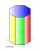0.7 l of water can be poured in an octagonal prism vase. What is the height of the vase, if the bottom has a area of 25 cm square and a thickness of 12 mm?
• Four prismsQuestion No. 1: The prism has the dimensions a = 2.5 cm, b = 100 mm, c = 12 cm. What is its volume? a) 3000 cm2 b) 300 cm2 c) 3000 cm3 d) 300 cm3 Question No.2: The prism base is a rhombus with a side length of 30 cm and a height of 27 cm. The height of t
• Sheet of paperIs the sheet of paper measuring 18 and 25 cm enough for taping 9 cm cubes with an edge? (1 = yes, 0 = no)
• Two rectangular boxesTwo rectangular boxes with dimensions of 5 cm, 8 cm, 10 cm, and 5 cm, 12 cm, 1 dm are to be replaced by a single cube box of the same cubic volume. Calculate its surface.
• Wooden bowls20 wooden bowls in the shape of a truncated cone should be painted on the outside and inside with wood varnish. We need 0.1 l of paint to paint 200 cm2. How many liters of paint do we have to buy if the bowls are 25 cm high, the bottom of the bowl has a d
• Water in aquariumThe aquarium cuboid shape with a length of 25 cm and a width of 30 cm is 9 liters of water. Calculate the areas which are wetted with water.
• Wall thicknessThe hollow metal ball has an outside diameter of 40 cm. Determine the wall thickness if the weight is 25 kg and the metal density is 8.45 g/cm3.
• Minimum surfaceFind the length, breadth, and height of the cuboid-shaped box with a minimum surface area, into which 50 cuboid-shaped blocks, each with length, breadth, and height equal to 4 cm, 3 cm, and 2 cm respectively, can be packed.
• Metal sheets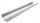How much metal sheet is needed to produce 8 gutters 4 m long and 12 cm in diameter? During production, joints calculate at 3% of total consumption.
• CapJesters hat is shaped by a rotating cone. Calculate how much paper is needed to the cap 54 cm high when the head circumference is 47 cm.
• Regular prismThe regular four-sided prism has a base of 25 cm2 and a surface of 210 cm2. Find its volume.
• Three cubesTwo cube-shaped boxes with edges a = 70 cm; b = 90 cm must be replaced by one cube-shaped box. What will be its edge?
• Metal rails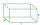Dad needs to improve the edges of the wooden boxes - to be reinforced with metal rails. How many cms of rails will he need if the box has the shape of a prism with the length of the edges 70cm 70cm and 120cm?
• TerezaThe cube has an area of base 256 mm2. Calculate the edge length, volume, and area of its surface.